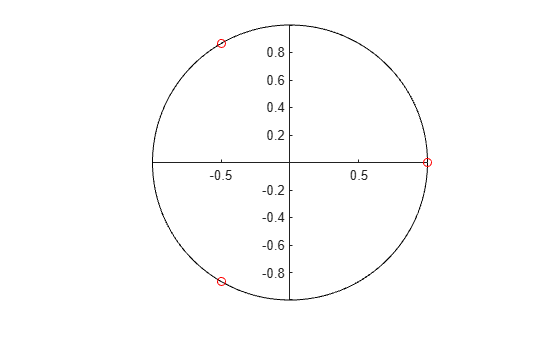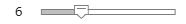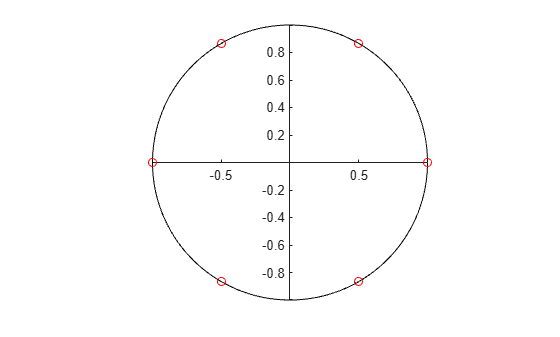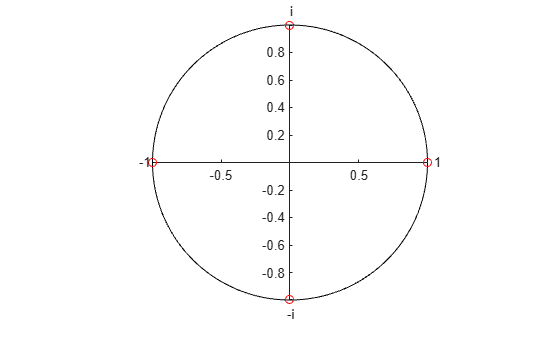# Create Interactive Course Materials Using the Live Editor

The following is an example of how to use live scripts in the classroom. This example shows how to:

• Add equations to explain the underlying mathematics.

• Execute individual sections of MATLAB code.

• Include plots for visualization.

• Use links and images to provide supporting information.

• Experiment with MATLAB code interactively.

• Reinforce concepts with other examples.

• Use live scripts for assignments.

### What does it mean to find the nth root of 1?

Add equations to explain the underlying mathematics for concepts that you want to teach. To add an equation, go to the Insert tab and click the Equation button. Then, select from the symbols and structures in the Equation tab.

Today we're going to talk about finding the roots of 1. What does it mean to find the nth root of 1? The nth roots of 1 are the solutions to the equation ${\mathit{x}}^{\mathit{n}}-1=0$.

For square roots, this is easy. The values are $\mathit{x}=±\sqrt{1}=±1$. For higher-order roots, it gets a bit more difficult. To find the cube roots of 1 we need to solve the equation ${\mathit{x}}^{3}-1=0$. We can factor this equation to get

`$\left(\mathit{x}-1\right)\left({\mathit{x}}^{2}+\mathit{x}+1\right)=0.$`

So the first cube root is 1. Now we can use the quadratic formula to get the second and third cube roots.

`$\mathit{x}=\frac{-\mathit{b}±\sqrt{{\mathit{b}}^{2}-4\mathrm{ac}}}{2\mathit{a}}$`

### Calculate the Cube Roots

To execute individual sections of MATLAB code, go to the Live Editor tab and click the Run Section button. Output appears together with the code that created it. Create sections using the Section Break button.

In our case a, b, and c are all equal to 1. The other two roots are calculated from these formulas:

```a = 1 ; b = 1 ; c = 1; roots = []; roots(1) = 1; roots(2) = (-b + sqrt(b^2 - 4*a*c))/(2*a); % Use the quadratic formula roots(3) = (-b - sqrt(b^2 - 4*a*c))/(2*a);```

So the full set of cube roots of 1 are:

`disp(roots')`
``` 1.0000 + 0.0000i -0.5000 - 0.8660i -0.5000 + 0.8660i ```

### Displaying Roots in the Complex Plane

Include plots in the Live Editor so students can visualize important concepts.

We can visualize the roots in the complex plane to see their location.

```range = 0:0.01:2*pi; plot(cos(range),sin(range),'k') % Plot the unit circle axis square; box off ax = gca; ax.XAxisLocation = 'origin'; ax.YAxisLocation = 'origin'; hold on plot(real(roots), imag(roots), 'ro') % Plot the roots```### Finding Higher Order Roots

To add supporting information, go to the Insert tab and click the Hyperlink and Image buttons. Students can use supporting information to explore lecture topics outside of the classroom.

Once you get past $n=3$, things get even trickier. For 4th roots we could use the quartic formula discovered by Lodovico Ferrari in 1540. But this formula is long and unwieldy, and doesn't help us find roots higher than 4. Luckily, there is a better way, thanks to a 17th century French mathematician named Abraham de Moivre.

Abraham de Moivre was born in Vitry in Champagne on May 26, 1667. He was a contemporary and friend of Isaac Newton, Edmund Halley, and James Stirling. https://en.wikipedia.org/wiki/Abraham_de_MoivreHe is best known for de Moivre's theorem that links complex numbers and trigonometry, and for his work on the normal distribution and probability theory. De Moivre wrote a book on probability theory, The Doctrine of Chances, said to have been prized by gamblers. De Moivre first discovered Binet's formula, the closed-form expression for Fibonacci numbers linking the nth power of the golden ratio φ to the nth Fibonacci number. He was also the first to postulate the Central Limit Theorem, a cornerstone of probability theory.

de Moivre's theorem states that for any real x and any integer n,

`${\left(\mathrm{cos}\text{\hspace{0.17em}}\mathit{x}+\mathit{i}\text{\hspace{0.17em}}\mathrm{sin}\text{\hspace{0.17em}}\mathit{x}\right)}^{\mathit{n}}=\mathrm{cos}\left(\mathrm{nx}\right)+\mathit{i}\text{\hspace{0.17em}}\mathrm{sin}\left(\mathrm{nx}\right).$`

How does that help us solve our problem? We also know that for any integer k,

`$1=\mathrm{cos}\left(2\mathit{k}\pi \right)+\mathit{i}\text{\hspace{0.17em}}\mathrm{sin}\left(2\mathit{k}\pi \right).$`

So by de Moivre's theorem we get

`${1}^{1/\mathit{n}}=\text{\hspace{0.17em}}{\left(\mathrm{cos}\left(2\mathit{k}\pi \right)+\mathit{i}\text{\hspace{0.17em}}\mathrm{sin}\left(2\mathit{k}\pi \right)\right)}^{1/\mathit{n}}=\mathrm{cos}\left(\frac{2\mathit{k}\pi }{\mathit{n}}\right)+\mathit{i}\text{\hspace{0.17em}}\mathrm{sin}\left(\frac{2\mathit{k}\pi }{\mathit{n}}\right).$`

### Calculating the nth Roots of 1

Use the Live Editor to experiment with MATLAB code interactively. Add controls to show students how important parameters affect the analysis. To add controls, go to the Live Editor tab, click the Control button, and select from the available options.

We can use this last equation to find the nth roots of 1. For example, for any value of n, we can use the formula above with values of $\mathit{k}=0\dots \mathit{n}-1$. We can use this MATLAB code to experiment with different values of n:

```n =6; roots = zeros(1, n); for k = 0:n-1 roots(k+1) = cos(2*k*pi/n) + 1i*sin(2*k*pi/n); % Calculate the roots end disp(roots')```
``` 1.0000 + 0.0000i 0.5000 - 0.8660i -0.5000 - 0.8660i -1.0000 - 0.0000i -0.5000 + 0.8660i 0.5000 + 0.8660i ```

Plotting the roots in the complex plane shows that the roots are equally spaced around the unit circle at intervals of $2\pi /\mathit{n}$.

```cla plot(cos(range),sin(range),'k') % Plot the unit circle hold on plot(real(roots),imag(roots),'ro') % Plot the roots```### Finding the nth roots of -1, i, and -i

Use additional examples to reinforce important concepts. Modify code during the lecture to answer questions or explore ideas in more depth.

We can find the roots of -1, i, and -i just by using extensions of the approach described above. If we look at the unit circle we see that the values of 1, i, -1, -i appear at angles $0$, $\pi /2$, $\pi$, and $3\pi /2$ respectively.

```r = ones(1,4); theta = [0 pi/2 pi 3*pi/2]; [x,y] = pol2cart(theta,r); cla plot(cos(range),sin(range),'k') % Plot the unit circle hold on plot(x, y, 'ro') % Plot the values of 1, i, -1, and -i text(x(1)+0.05,y(1),'1') % Add text labels text(x(2),y(2)+0.1,'i') text(x(3)-0.1,y(3),'-1') text(x(4)-0.02,y(4)-0.1,'-i')```Knowing this, we can write the following expression for i:

`$\mathit{i}=\mathrm{cos}\left(\left(2\mathit{k}+1/2\right)\pi \right)+\mathit{i}\text{\hspace{0.17em}}\mathrm{sin}\left(\left(2\mathit{k}+1/2\right)\pi \right).$`

Taking the nth root of both sides gives

`${\mathit{i}}^{1/\mathit{n}}={\left(\mathrm{cos}\left(\left(2\mathit{k}+1/2\right)\pi \right)+\mathit{i}\text{\hspace{0.17em}}\mathrm{sin}\left(\left(2\mathit{k}+1/2\right)\pi \right)\right)}^{1/\mathit{n}}$`

and by de Moivre's theorem we get

`${\mathit{i}}^{1/\mathit{n}}={\left(\mathrm{cos}\left(\left(2\mathit{k}+1/2\right)\pi \right)+\mathit{i}\text{\hspace{0.17em}}\mathrm{sin}\left(\left(2\mathit{k}+1/2\right)\pi \right)\right)}^{1/\mathit{n}}=\mathrm{cos}\left(\frac{\left(2\mathit{k}+1/2\right)\pi }{\mathit{n}}\right)+\mathit{i}\text{\hspace{0.17em}}\mathrm{sin}\left(\frac{\left(2\mathit{k}+1/2\right)\pi }{\mathit{n}}\right).$`

### Homework

Use live scripts as the basis for assignments. Give students the live script used in the lecture and have them complete exercises that test their understanding of the material.

Use the techniques described above to complete the following exercises:

Exercise 1: Write MATLAB code to calculate the 3 cube roots of i.

`% Put your code here`

Exercise 2: Write MATLAB code to calculate the 5 fifth roots of -1.

`% Put your code here`

Exercise 3: Describe the mathematical approach you would use to calculate the nth roots of an arbitrary complex number. Include the equations you used in your approach.